# RRR: A Succinct Rank/Select Index for Bit Vectors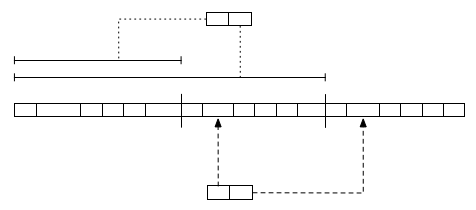This blog post will give an overview of a static bitsequence data structure known as RRR, which answers arbitrary length rank queries in $\mathcal{O}(1)$ time, and provides implicit compression.

As my blog is informal, I give an introduction to this structure from a birds eye view. If you want, read my thesis for a version with better markup, and follow the citations for proofs by people smarter than myself :)

My intended future posts will cover the other aspects of my thesis, including generalising RRR (for sequences over small alphabets), Wavelet Trees (which answer rank queries over bigger alphabets), and Suffix Arrays (a text index which – when combined with the above structures – can answer queries in $\mathcal{O}(P \log_2 A)$ time, when $P$ is the length of the search pattern, and $A$ is the alphabet size).

Update: I have now posted about Wavelet Trees! Check it out here.

## Example Problem

Cracking the Oyster, the first column of Programming Pearls, opens with a programmer asking for advice when sorting around ten million unique seven-digit integers – phone numbers.

After some discussion, the author concludes that a bitmap should be used. If we wanted to store ten million integers, we could use an array of $32$-bit integers, consuming $38$ MB, or we could represent our numbers as positions on a number line.

All of these phone numbers will be within the range $[0000000, 9999999]$. To represent the presence of these numbers, we only need a bitmap $10^7$ bits long, about $1$ MB, which would represent our number line. Then, for a bitmap $M$, if we want to store phone number $p$, we set the bit $M[p]$ to $1$. Sorting would involve setting the numbers that are present to $1$, then iterating over the bitmap, printing the positions of the $1$-bits – $\mathcal{O}(N)$ time.

In the following sections, I will detail operations that can be done on bitmaps, named rank and select, and explain how to answer rank queries in $\mathcal{O}(1)$ time, and implicitly compress the bitmap. Using rank and select, a compressed bitmap can be a very powerful way to store sets. This isn’t limited to just sets of numbers, all sorts of things, such as tree or graph nodes for example.

## Extension: Rank

Allow me to extend the problem. I want to query our simple phone number database to see how many phone numbers are allocated within the range $[0005000, 0080000]$. I could iterate over that range and update a counter whenever I encounter a $1$-bit. Actually, this operation is what is known as a rank operation.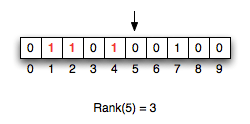The operation $rank(i)$ is defined as the number of set bits ($1$s) in the range $[0, i]$ (or $[0, i)$ in some papers). In the bitstring above, the answer to $rank(5)$ is $3$… This is a generalisation of the popcount operation which counts all set bits, which I have discussed before (here and here). $rank(i)$ can be implemented by left-shifting $L – i$ bits (where $L$ is the length of the datatype you are using, int, long, etc) to remove the unwanted bits, then calling $popcount$ on the resulting value. This could be done iteratively over an array if you want, but I will discuss a much faster way below.

Then, the above question can be answered as: $rank(0080000) – rank(0005000 – 1)$. This will give us just the number of $1$s between $0005000$ and $0080000$.

This isn’t the only place we would use a popcounts; it happens that popcounts are common enough that we want to optimise them. Check out this blog post at valuedlessons.com for a discussion and empirical comparison of several fast approaches.

## RRR

As it happens, we can build a data structure for static bitmaps that answers rank queries in $\mathcal{O}(1)$ time, and provides implicit compression. It is what is known as a succinct data structure, which means that even though it is compressed, we don’t need to decompress the whole thing t operate on it efficiently. Sadakane (a respected researcher in succinct data structures) gives a nice analogy in his introduction of the field, likening it to forcing dehydrated noodles apart with your chopsticks (decompression) as you are rehydrating them, but before the whole thing is fully cooked and separated. This allows you to keep some of the noodles compressed while you eat the decompressed fragment.

Since it is static it isn’t well suited for a bitmap which you want to update (although work has been done toward this), it is still really cool :)

The structure I’m referring to is named RRR. It sounds like a radio station, but it is named after its creators: Raman, Raman, Rao, from their 2002 paper Succinct indexable dictionaries with applications to encoding $k$-ary trees and multisets. Its a data structure I had to become intimately involved with for my honours thesis, where I extended it for sequences of larger (but still small) alphabets. If you want to answer rank queries on large alphabets, a wavelet tree might be what you are after, but that will be covered in a different blog post (or you could read my thesis!).

In my last post (Generating Binary Permutations in Popcount Order) I discussed how to compress a bitstring by replacing blocks of a certain blocksize with their corresponding pop number, and (variable length) offset into a lookup table. I briefly mentioned building an index over it to improve lookup as well.

## RRR: Construction

To construct a RRR sequence we divide our bitmap into blocks, as I mentioned in my previous blog post. These are grouped in superblocks, too, which allows us to construct an index to enable $\mathcal{O}(1)$ rank queries. In the following image, I have fragmented the bitmap using a blocksize of $b = 5$, and grouped them with a superblock factor of $f = 3$ – so each superblock is three blocks.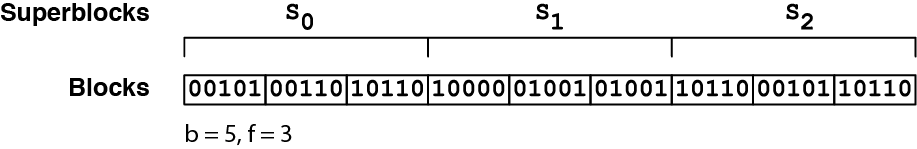First we replace the blocks with a pair of values, a class value $C$ and offset value $O$, which are used together as a lookup key into a table of precomputed (small – for each possible block only) ranks – this is demonstrated in the figure below. This is the same as the previous blog post, although in that I called the “class” $P$. This is because the class of a block is defined as the popcount – the number of set bits – in the block: $class(B) = popcount(B)$ for block $B$.The table is shared among all your RRR sequences, and is in fact a table of tables, where $C$ points to the first element for the ranks of a given popcount:For this table (let’s call it $G$), for a given class $C$, the sub-table at $G[C]$ has $b \choose C$ entries, which correspond to all possible permutations that have a popcount of $C$. This means that while our $C$ values will always be $\log{b + 1}$ (the number of bits to represent values $0, 1, 2… b$ – these are all possible popcount values for the blocksize), but our $O$ values will vary in size, requiring $\log{b \choose C}$ bits (oh yeah, and of course I’m using $\log_2$ here :)). During a query, we can use our $C$ values to work out how many bits will follow for the $O$ values.

Using this approach alone we get the compression, but not $\mathcal{O}(1)$ ranks. $C$ is fixed width, the compression comes from $O$ being varied width.

In order to get the $\mathcal{O}(1)$ ranks we use a method discussed by Munro in Tables, 1996. This is where the superblocks come in to play: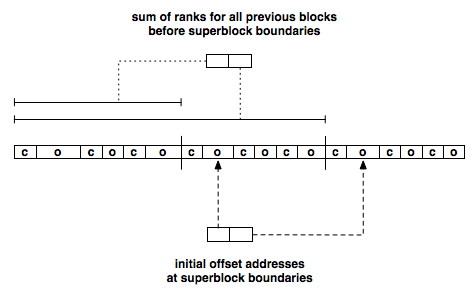For each superblock boundary we store the global rank up to that position. We also store a prefix sum of the bits, which gives us the address to the first block in the next superblock (since it is variable length!). This allows us to not require iterating over the whole RRR sequence, but instead going straight to the required superblock. We will only need to iterate over the blocks within a superblock, so it is now bound by whatever your superblock factor is.

## RRR: Querying

To calculate $rank(i)$:

1. Calculate which block our index is in as $i_b = \frac{i}{b}$. ($i_b$ is the global index of the block)
2. Calculate which superblock our block resides in as $i_s = \frac{i_b}{f}$. ($i_s$ is the index of the superblock)
3. Set result to the sum of previous ranks at is boundary (which is pre-calculated).
4. Using each blocks class-offset pair $(c,o)$ after the boundary at is, add the rank for that entire block to result.
5. Repeat previous step until we reach $i_b$. We then add $rank(j,c)$ (from $i_b$, not the global rank) to our result, where $j = i \mod b$, and is the position we are querying local to $i_b$. Our final answer is the result.

## Select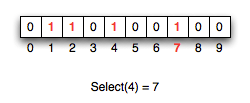Select is the inverse operation to rank; it answers the question “at which position is the $i^{th}$ set bit?”. To tie this in with the phone numbers example, maybe we want to find out the fiftieth phone number in the set (excluding unassigned numbers). This is a way we can index just the present elements of a bitmap. It turns out select can be answered in $\mathcal{O}(1)$ time as well. I won’t cover select here, as my future posts (and thesis) will mainly use rank. You can read about it in the RRR paper.

## Go Forth and…

Feel free to implement this (somewhat complicated) data structure yourself, or you can use a pre-rolled one by my friend Francisco Claude in his LIBCDS – Compressed Data Structure Library.

algorithmbinarycompressionsuccinctdata structures

Alex has a PhD in succinct data structures for bioinformatics, and currently works on self-driving cars. He's also interested in cryptocurrency, business, fashion, photography, teaching, and writing.

## Succinct de Bruijn Graphs

This post will give a brief explanation of a Succinct implementation for storing de Bruijn graphs [http://en.wikipedia.org/wiki/De_Bruijn_graph], which is recent (and continuing) work I have been doing with Sadakane. Using our new structure, we have squeezed a graph for a human genome (which

## FM-Indexes and Backwards Search

Last time (way back in June! I have got to start blogging consistently again) I discussed a gorgeous data structure called the Wavelet Tree [https://alexbowe.com/wavelet-trees/]. When a Wavelet Tree is stored using RRR sequences, it can answer rank and select operations in $\mathcal{O}(\log{A})$ time,

## Wavelet Trees: an Introduction

Today I will talk about an elegant way of answering rank queries on sequences over larger alphabets – a structure called the Wavelet Tree. In my last post [https://alexbowe.com/yarrr-me-hearties] I introduced a data structure called RRR, which is used to quickly answer rank queries on binary sequences, and